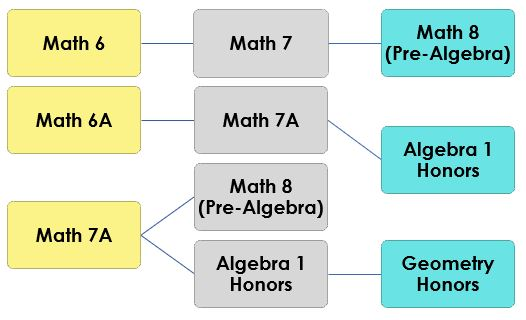# MathIn grade 6, instructional time will emphasize five areas: (1) performing all four operations with integers, positive decimals and positive fractions with procedural fluency; (2) exploring and applying concepts of ratios, rates and percent to solve problems; (3) creating, interpreting and using expressions and equations; (4) extending geometric reasoning to plotting points on the coordinate plane, area and volume of geometric figures and (5) extending understanding of statistical thinking.

In grade 6 accelerated, instructional time will emphasize five areas: (1) performing all four operations with rational numbers with procedural fluency; (2) exploring and applying concepts of ratios, rates, percentages and proportions to solve problems; (3) creating, interpreting and using expressions, equations and inequalities; (4) extending geometric reasoning to plotting points on the coordinate plane, area and volume of geometric figures and (5) extending understanding of statistical thinking to represent and compare categorical and numerical data.

In grade 7, instructional time will emphasize five areas: (1) recognizing that fractions, decimals and percentages are different representations of rational  numbers  and  performing all  four  operations with rational numbers with procedural fluency; (2) creating equivalent expressions and solving equations and inequalities; (3) developing understanding of and applying proportional relationships in two variables; (4) extending analysis of two- and three-dimensional figures to include circles and cylinders and (5) representing and comparing categorical and numerical data and developing understanding of probability.

In grade 7 accelerated, instructional time will emphasize six areas: (1) representing numbers in scientific notation and extending the set of numbers to the system of real numbers, which includes irrational numbers; (2) generating equivalent numeric and algebraic expressions including using the Laws of Exponents; (3) creating and reasoning about linear relationships including modeling an association in bivariate data with a linear equation; (4) solving linear equations, inequalities and systems of linear equations; (5) developing an understanding of the concept of a function and (6) analyzing two-dimensional figures, particularly triangles, using distance, angle and applying the Pythagorean Theorem.

Algebra 1 Honors

In Algebra 1 Honors, instructional time will emphasize five areas: (1) performing operations with polynomials and radicals, and extending the Laws of Exponents to include rational exponents; (2) extending understanding of functions to linear, quadratic and exponential functions and using them to model and analyze real-world relationships; (3) solving quadratic equations in one variable and systems of linear equations and inequalities in two variables; (4) building functions, identifying their key features and representing them in various ways and (5) representing and interpreting categorical and numerical data with one and two variables.